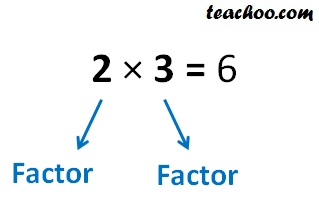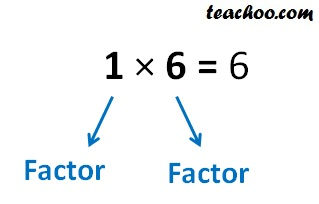Factors and Multiples

Chapter 3 Class 6 Playing with Numbers
Concept wise

Suppose we multiply two numbers to get a numberHere,

2 & 3 are factors of 6

Similarly,Here,

1 & 6 are factors of 6

## How to find factors?

Suppose we have to find factors of 6

• We start with 1, and multiply it with a number to get 6.
• Then, we do the same with 2, 3, 4, 5.. until the number repeats

So,

1 × 6 = 6

2 × 3 = 6

3 × 2 = 6

We stop here as 3, 2 have occurred earlier

∴ Factors of 6 are 1, 2, 3, 6

### Find factors of 8

1 × 8 = 8

2 × 4 = 8

3 ×

4 × 2 = 8

We stop here as 2 & 4 have occurred earlier

So, factors of 8 are 1, 2, 4, 8

### Find factors of 14

1 × 14 = 14

2 × 7 = 14

3 ×

4 ×

5 ×

6 ×

7 × 2 = 14

We stop here as 2 & 7 have occurred earlier

So, factors of 14 are 1, 2, 7, 14

### Find factors of 30

1 × 30 = 30

2 × 15 = 30

3 × 10 = 30

4 ×

5 × 6 = 30

6 ×  5 = 30

We stop here as 5 & 6 have occurred earlier

So, factors of 30 are 1, 2, 3, 5, 6, 10, 15, 30

## Properties of factor

• 1 is the factor of every number
• Every number is the factor of itself
• Every factor is less than or equal to the given number
• There are finite number of factors

Learn in your speed, with individual attention - Teachoo Maths 1-on-1 Class